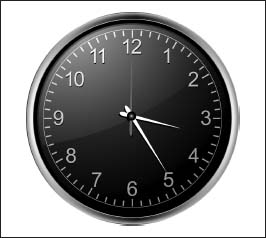NextPrevious

# What is the Fibonacci sequence?We can look to the familiar face of a clock to illustrate the concept of “clock arithmetic” or modulo 12. With clocks, we can count through 11 before getting to 12, where we start over again at zero. Thus, if it is 7 o’clock and we add 6 hours, we get 1 o’clock, not 13 o’clock.

Italian mathematician Leonard of Pisa (c. 1170-c. 1250; also known as Fibonacci, or “son of Bonacci,” although some historians say there is no evidence that he or his contemporaries ever used the name) may be known for helping to introduce Hindu-Arabic numerals to Europe (for more information about Fibonacci and numerals, see elsewhere in this chapter), but he also is famous for the sequence of numbers he discovered. This sequence—initially pursued as an exercise to determine how fast a pair of rabbits can reproduce per year—is formed by adding the two preceding numbers to find the next number, starting with a pair of ones. Thus, the Fibonacci numbers in this sequence are 1, 1, 2, 3, 5, 8, 13, 21, 34, 55, 89, 144, 233, 377, and so on; or

1 + 1 = 2

2 + 1 = 3

3 + 2 = 5

5 + 3 = 8

8 + 5 = 13

13 + 8 = 21

21 + 13 = 34

34 + 21 = 55

55 + 34 = 89

89 + 55 = 144

144 + 89 = 233

233 + 144 = 377, and so on.

Close

This is a web preview of the "The Handy Math Answer Book" app. Many features only work on your mobile device. If you like what you see, we hope you will consider buying. Get the App